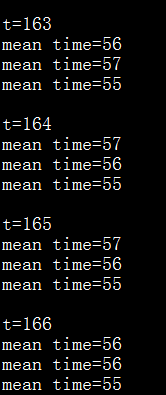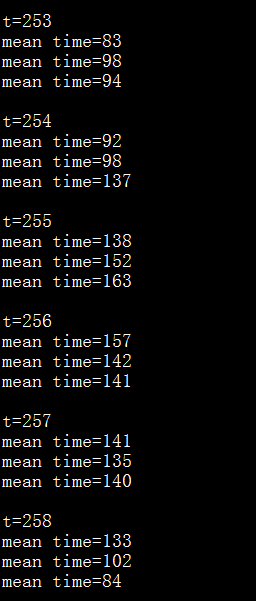Re: [eigen] Different computation time when using Eige
• To: eigen@xxxxxxxxxxxxxxxxxxx
• Subject: Re: [eigen] Different computation time when using Eige
• From: tuch s <tuchfly@xxxxxxxxx>
• Date: Mon, 27 Jun 2016 18:42:40 +0800
• Dkim-signature: v=1; a=rsa-sha256; c=relaxed/relaxed; d=gmail.com; s=20120113; h=mime-version:in-reply-to:references:from:date:message-id:subject:to; bh=HWEcgLoYJA6aInViRDq7f9B1aVpXiVQDoX4qnjiBTmM=; b=RqjUQ3h5b1LjjpPXjWRESZnFTT4t1GhEKNB3vXu+YnaHkUU+MEPeV76tsEEmyo30Rn S1mE3WYB1YwhntnsXeIQx5zUL64PPWXJx9cEg700ksTj5rF2lMD7L3UtKn8duALL21ek 4+FrYgestbYoUoIlL2ctOBFinlM8xRK5O3RZe5KfoSRLrTyfg1EbPiyoadLNM6/5EyrL DS15z+1r1bYxt8bTwhHZSSIVq+pfmdxB50sI2r/q+ePEoA5OEIlYSx7S4aNB8QMNnCt2 vNk7FDu61R8VnZt2THpCVG+YhjM/lOP3rxuorS6MH/SpNCyofTnPDBYjuQVq05SDKx0d Acfg==

here is the running time using this example:On Mon, Jun 27, 2016 at 6:32 PM, tuch s wrote:
Acturally, I was running this code online:

most of the time was cost by this function:

void LSTM::forward(const VecD& xt, const LSTM::State* prev, LSTM::State* cur){
cur->i = this->bi;
cur->i.noalias() += this->Wxi*xt + this->Whi*prev->h;
cur->f = this->bf;
cur->f.noalias() += this->Wxf*xt + this->Whf*prev->h;
cur->o = this->bo;
cur->o.noalias() += this->Wxo*xt + this->Who*prev->h;
cur->u = this->bu;
cur->u.noalias() += this->Wxu*xt + this->Whu*prev->h;

ActFunc::logistic(cur->i);
ActFunc::logistic(cur->f);
ActFunc::logistic(cur->o);
ActFunc::tanh(cur->u);
cur->c = cur->i.array()*cur->u.array() + cur->f.array()*prev->c.array();
cur->cTanh = cur->c;
ActFunc::tanh(cur->cTanh);
cur->h = cur->o.array()*cur->cTanh.array();
}

I have created a sample example, the running time is also some kind of different for the same data, but I am not sure is it the same for above function.

#include <iostream>
#include <Eigen/Dense>
#include <Eigen/Core>
#include <sys/time.h>

using Eigen::MatrixXd;
using namespace Eigen;
using namespace Eigen::internal;
using namespace Eigen::Architecture;
using namespace std;

long long myTime()
{
struct timeval tm;
gettimeofday(&tm, NULL);
return tm.tv_sec*1000*1000 + tm.tv_usec;
}

int main(int argc, char* argv[])
{
MatrixXd m1(128, 1024), m2(128, 1024), m3(128, 1024), m4(128, 1024);

VectorXd v1(1024), v2(1024), v3(1024), v4(1024);

for(int j = 0; j < 1024; ++j)
{
v1(j) = j * 0.234 + 1;
v2(j) = j * 0.134 + 0.3;
v3(j) = j * 0.124 + 0.7;
v4(j) = j * 0.034 + 0.5;
for(int i = 0; i < 128; ++i)
{
m1(i, j) = i*0.378 + j*0.024 + 3.2;
m2(i, j) = i*1.384 + j*0.124 + 0.2;
m3(i, j) = i*0.778 + j*0.004 + 1.2;
m4(i, j) = i*0.538 + j*1.024 + 0.7;
}
}

for(int t = 0; t < 1000; ++t)
{
cout <<"\nt="<<t<<endl;
VectorXd x(128), y(128);

long long tm0 = myTime();
for(int i = 0; i < 10; ++i)
{
x = v1;
x += m1 * v1 + m2 * v2;

y = v3;
y += m3 * v3 + m4 * v4;
}
long long tm1 = myTime();
cout <<"mean time="<<(tm1-tm0)/1000<<endl;

long long tm3 = myTime();
for(int i = 0; i < 10; ++i)
{
x = v1;
x.noalias() += m1 * v1 + m2 * v2;

y = v3;
y.noalias() += m3 * v3 + m4 * v4;
}
long long tm4 = myTime();
cout <<"mean time="<<(tm4-tm3)/1000<<endl;

for(int i = 0; i < 10; ++i)
{
x = v1;
x.noalias() += m1 * v1;
x.noalias() += m2 * v2;

y = v3;
y.noalias() += m3 * v3;
y.noalias() += m4 * v4;
}
long long tm5 = myTime();
cout <<"mean time="<<(tm5-tm4)/1000<<endl;

}

}

On Mon, Jun 27, 2016 at 6:18 PM, tuch s wrote:
The eigen version is  Eigen 3.2.8

On Mon, Jun 27, 2016 at 6:15 PM, tuch s wrote:
The input data and output date are the same, but the running time is much different, some times very fast, some times much slower.

The matrix is 128*1024, and the vector is 1024, I didn't know why the running time is so much different.

0.4 second and 2.1 second is just an example, maybe this time I can get the output using just 0.4 second, and the next time 1.9 second, or 2.1 second or some other value.

On Mon, Jun 27, 2016 at 5:54 PM, Christoph Hertzberg wrote:
Hi!

I'd need a bit more details to get any idea what is happening. E.g.:
Are the matrix sizes the same all the time (and how big are they)?
Does cur->i = this->bi involve resizing/memory allocation?
Is 0.4--2.1 seconds the time for all for computations or for each (or for multiple times)?
What Eigen version are you running?

The easiest way for us would be, if you could produce a minimal working example which exposes the problem.

Cheers,
Christoph

On 2016-06-27 11:37, tuch s wrote:
I have used eigen to do the following computation in a deep learning
programme，
Code: Select all
<https://forum.kde.org/viewtopic.php?f=74&t=133580&p=359022#>  cur->i =
this->bi;
cur->i.noalias() += this->Wxi*xt + this->Whi*prev->h;
cur->f = this->bf;
cur->f.noalias() += this->Wxf*xt + this->Whf*prev->h;
cur->o = this->bo;
cur->o.noalias() += this->Wxo*xt + this->Who*prev->h;
cur->u = this->bu;
cur->u.noalias() += this->Wxu*xt + this->Whu*prev->h;

W* is a matrix, and h, f, i, o, u are all vectors.

But I tried several times with the same input data， the time consuming is
much different.
Some times it costs 0.4 second to get the output, but some times it cost
2.1 second to get the output.

How can I solve this problem to get stable time cost ?

--
Dipl. Inf., Dipl. Math. Christoph Hertzberg

Universität Bremen
FB 3 - Mathematik und Informatik
AG Robotik
Robert-Hooke-Straße 1
28359 Bremen, Germany

Zentrale: +49 421 178 45-6611

Robert-Hooke-Straße 5
28359 Bremen, Germany

Tel.:    +49 421 178 45-4021
Empfang: +49 421 178 45-6600
Fax:     +49 421 178 45-4150
E-Mail:  chtz@xxxxxxxxxxxxxxxxxxxxxxxx

Weitere Informationen: http://www.informatik.uni-bremen.de/robotik

 Mail converted by MHonArc 2.6.19+ http://listengine.tuxfamily.org/